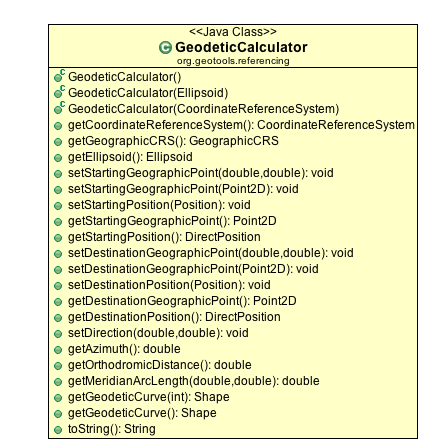# GeodeticCalculator¶

The GeodeticCalculator is used to perform calculations on the Geoid (i.e. on the surface of the world).You can configure your GeodeticCalculator to work with a specific Ellipsoid (or commonly from an CoordinateReferenceSystem) and then use it to perform a number

References:

## Distance¶

• Distance between two points

```        // the following code is based on JTS.orthodromicDistance( start, end, crs )
GeodeticCalculator gc = new GeodeticCalculator(crs);
gc.setStartingPosition(JTS.toDirectPosition(start, crs));
gc.setDestinationPosition(JTS.toDirectPosition(end, crs));

double distance = gc.getOrthodromicDistance();

int totalmeters = (int) distance;
int km = totalmeters / 1000;
int meters = totalmeters - (km * 1000);
float remaining_cm = (float) (distance - totalmeters) * 10000;
remaining_cm = Math.round(remaining_cm);
float cm = remaining_cm / 100;

System.out.println("Distance = " + km + "km " + meters + "m " + cm + "cm");
```

Although the above shows quickly creating a DirectPosition from a JTS Coordinate; you can use the GeodedicCalculator with any two positions, internally it will transform the points as needed.

• You can also generate the angle between the two points

Continuing on from the previous example:

```        double angle = gc.getAzimuth();

System.out.println("Angle = " + angle);
```
• Generate location away from a point

Finally you can turn the tables and use the GeodeticCalculator to generate a point a set direction and distance away from your starting point.

```        GeodeticCalculator calc = new GeodeticCalculator();
// mind, this is lon/lat
calc.setStartingGeographicPoint(45.4644, 9.1908);
calc.setDirection(90 /* azimuth */, 200 /* distance */);
Point2D dest = calc.getDestinationGeographicPoint();
System.out.println("Longitude: " + dest.getX() + " Latitude: " + dest.getY());
```